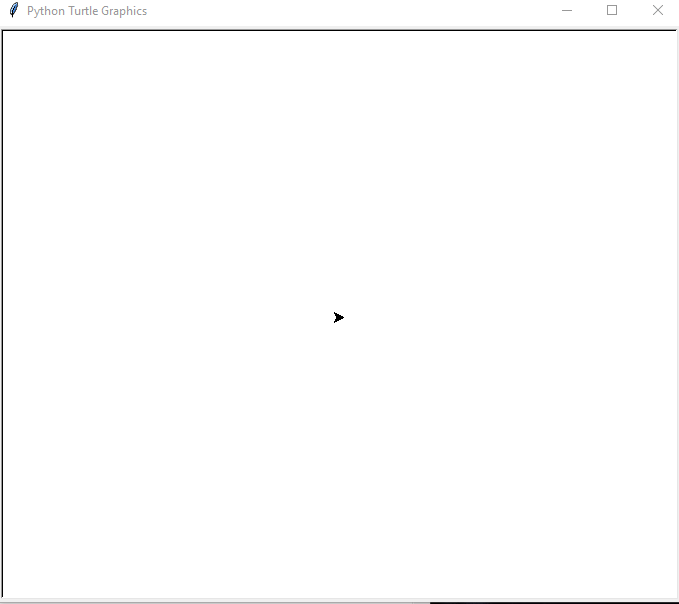# How to make Triangle in Python Turtle using onscreenclick?

• Last Updated : 11 Jun, 2021

Turtle” is a Python feature like a drawing board, which lets us command a turtle to draw all over it! We can use functions like turtle.forward(…) and turtle.right(…) which can move the turtle around. Turtle is also Known as Logo Programming language which commands for movement and drawing produced line or vector graphics, either on screen or with a small robot termed a turtle.

#### Functions used

• Turtle(): This Method is used to make object.
• onscreenclick(functionname,1):  This turtle function which sends the current coordinate to function which further utilise to form triangle, 1 is for left click and 3 is for Right click
• speed(): This is used to increase or decrease the speed of turtle pointer.
• listen(): This allows the server to listen to incoming connections.
• done(): This is used to hold the the screen.
• penup(): This is built in function in turtle library to draw out the line.
• pendown(): This is built in function in turtle library to draw in the line.
• forward(): This is built in function in turtle library to move turtle forward direction.it takes pixel unit as an argument
• left(): This is built in function in turtle library to turn turtle left direction.it takes angle in degree as an argument

## Python3

 `import` `turtle`  `# Screen() method to get screen``wn``=``turtle.Screen() ` `# creating tess object``tess``=``turtle.Turtle() `  `def` `triangle(x,y):``  ` `    ``# it is used to draw out the pen``    ``tess.penup()``    ` `    ``# it is used to move cursor at x``    ``# and y position``    ``tess.goto(x,y)``    ` `    ``# it is used to draw in the pen``    ``tess.pendown()``    ``for` `i ``in` `range``(``3``):``      ` `          ``# move cursor 100 unit``        ``# digit forward``        ``tess.forward(``100``)``        ` `        ``# turn cursor 120 degree left``        ``tess.left(``120``)``        ` `        ``# Again,move cursor 100 unit``        ``# digit forward``        ``tess.forward(``100``)``        ` `# special built in function to send current``# position of cursor to triangle``turtle.onscreenclick(triangle,``1``)` `turtle.listen()` `# hold the screen``turtle.done()`

Output :As we can see cursor forms triangle where we can

My Personal Notes arrow_drop_up